# Prime and Composite Numbers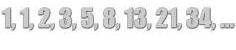Problem 1: The area of a rectangular garden is 7 square yards. List all possible whole-number dimensions the garden can have.

Solution: 1 yd x 7 yd

The whole-number dimensions, 1 and 7, of the rectangular garden above, are the factors of the number 7.

Problem 2: The area of a rectangular garden is 8 square yards. List all possible whole-number dimensions the garden can have.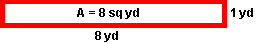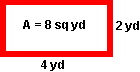Solution: 1 yd x 8 yd,  2 yd x 4 yd

The whole-number dimensions, 1, 2, 4 and 8, of the rectangular gardens in Problem 2, are the factors of the number 8. In Problem 1, the number 7 has only two factors. The number 7 is prime. In problem 2 above, the number 8 has four factors. The number 8 is composite.

#### Definitions

1. prime number has only two factors: 1 and itself.
2. composite number has more than two factors.
3. The number 1 is neither prime nor composite.

When the area of a rectangle is a prime number, there is only one set of possible dimensions for that rectangle. When the area of a rectangle is a composite number, there are two or more sets of possible dimensions for that rectangle. Each set of dimensions is a pair of factors.

To determine if a number is prime or composite, follow these steps:

1. Find all factors of the number.
2. If the number has only two factors, 1 and itself, then it is prime.
3. If the number has more than two factors, then it is composite.

Example 1: Is the number 2 prime or composite?

Solution: The factors of 2 are 1 x 2. 2 is prime.

Example 2: Is the number 9 prime or composite?

Solution: The factors of 9 are 1 x 9, 3 x 3. 9 is composite.

We have determined if a single number is prime or composite. Let's look at a range of numbers to see if they are prime or composite. Please note that each range of numbers given in Examples 3, 4 and 5 below are inclusive.

Example 3: Find all prime numbers between 2 and 9.

 factors of 2: 1 x 2 2 is prime factors of 3: 1 x 3 3 is prime factors of 4: 1 x 4, 2 x 2 4 is composite factors of 5: 1 x 5 5 is prime factors of 6: 1 x 6, 2 x 3 6 is composite factors of 7: 1 x 7 7 is prime factors of 8: 1 x 8, 2 x 4 8 is composite factors of 9: 1 x 9, 3 x 3 9 is composite

Solution: The prime numbers between 2 and 9 are 2, 3, 5 and 7.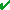Example 4: Find all prime numbers between 10 and 19.

 factors of 10: 1 x 10, 2 x 5 10 is composite factors of 11: 1 x 11 11 is prime factors of 12: 1 x 12, 2 x 6, 3 x 4 12 is composite factors of 13: 1 x 13 13 is prime factors of 14: 1 x 14, 2 x 7 14 is composite factors of 15: 1 x 15, 3 x 5 15 is composite factors of 16 1 x 16, 4 x 4 16 is composite factors of 17: 1 x 17 17 is prime factors of 18: 1 x 18, 3 x 6 18 is composite factors of 19: 1 x 19 19 is prime

Solution: The prime numbers between 10 and 19 are 11, 13, 17 and 19.Example 5: Find all prime numbers between 20 and 29.

 factors of 20: 1 x 20, 2 x 10, 4 x 5 20 is composite factors of 21: 1 x 21, 3 x 7 21 is composite factors of 22: 1 x 22, 2 x 11 22 is composite factors of 23: 1 x 23 23 is prime factors of 24: 1 x 24, 2 x 12, 3 x 8, 4 x 6 24 is composite factors of 25: 1 x 25, 5 x 5 25 is composite factors of 26: 1 x 26, 2 x 13 26 is composite factors of 27: 1 x 27, 3 x 9 27 is composite factors of 28: 1 x 28, 2 x 14, 4 x 7 28 is composite factors of 29: 1 x 29 29 is prime

Solution: The prime numbers between 20 and 29 are 23 and 29.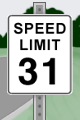Example 6: Is the number 31 prime or composite? Explain your answer using full sentences.

Solution 1: The number 31 is prime because its only factors are one and itself.

Solution 2: Thirty-one is a prime number. This is because the number 31 has only two factors: 1 and 31.

Solution 3: I divided the number 31 by all numbers between 1 and 31 and found no factors other than one and thirty-one. Therefore, 31 is prime.

There are many possible ways to explain the solution to this problem. These are just three possible explanations.

Summary: A prime number has only two factors: 1 and itself. A composite number has more than two factors. The number 1 is neither prime nor composite.

The prime numbers between 2 and 31 are 2, 3, 5, 7, 11, 13, 17, 19, 23, 29 and 31 since each of these numbers has only two factors, itself and 1.

### Exercises

Directions: Read each question below. Select your answer by clicking on its button. Feedback to your answer is provided in the RESULTS BOX. If you make a mistake, choose a different button.

 1 Each of the following numbers is composite EXCEPT:30, 31, 32, 33, 34, 35, 36, 37, 38, 39 32 and 3930 and 3431 and 37All composite RESULTS BOX:
 2 The prime numbers between 40 and 49 are: 42, 43 and 4741, 43 and 47 43, 45 and 47None of the above. RESULTS BOX:
 3 The prime numbers between 50 and 59 are: 53 and 5951 and 59 53 and 57None of the above. RESULTS BOX:
 4 The prime numbers between 60 and 69 are: 63 and 6961 and 67 60 and 65None of the above. RESULTS BOX:
 5 The prime numbers between 20 and 69 are: 21, 23, 29, 31, 37, 41, 43, 47, 53, 63 and 6923, 29, 31, 33, 37, 41, 43, 47, 59, 61 and 6723, 29, 31, 37, 41, 43, 47, 53, 59, 61 and 67None of the above. RESULTS BOX: